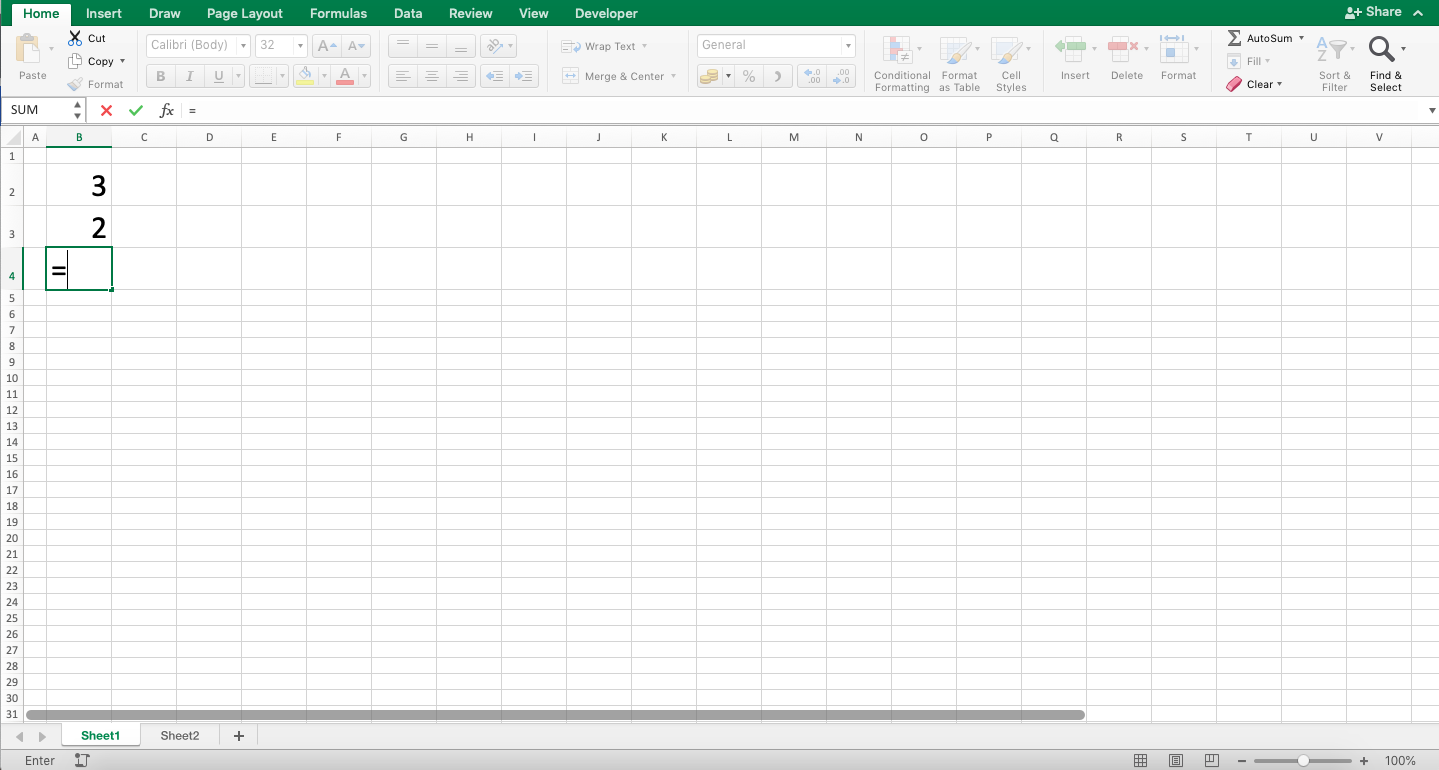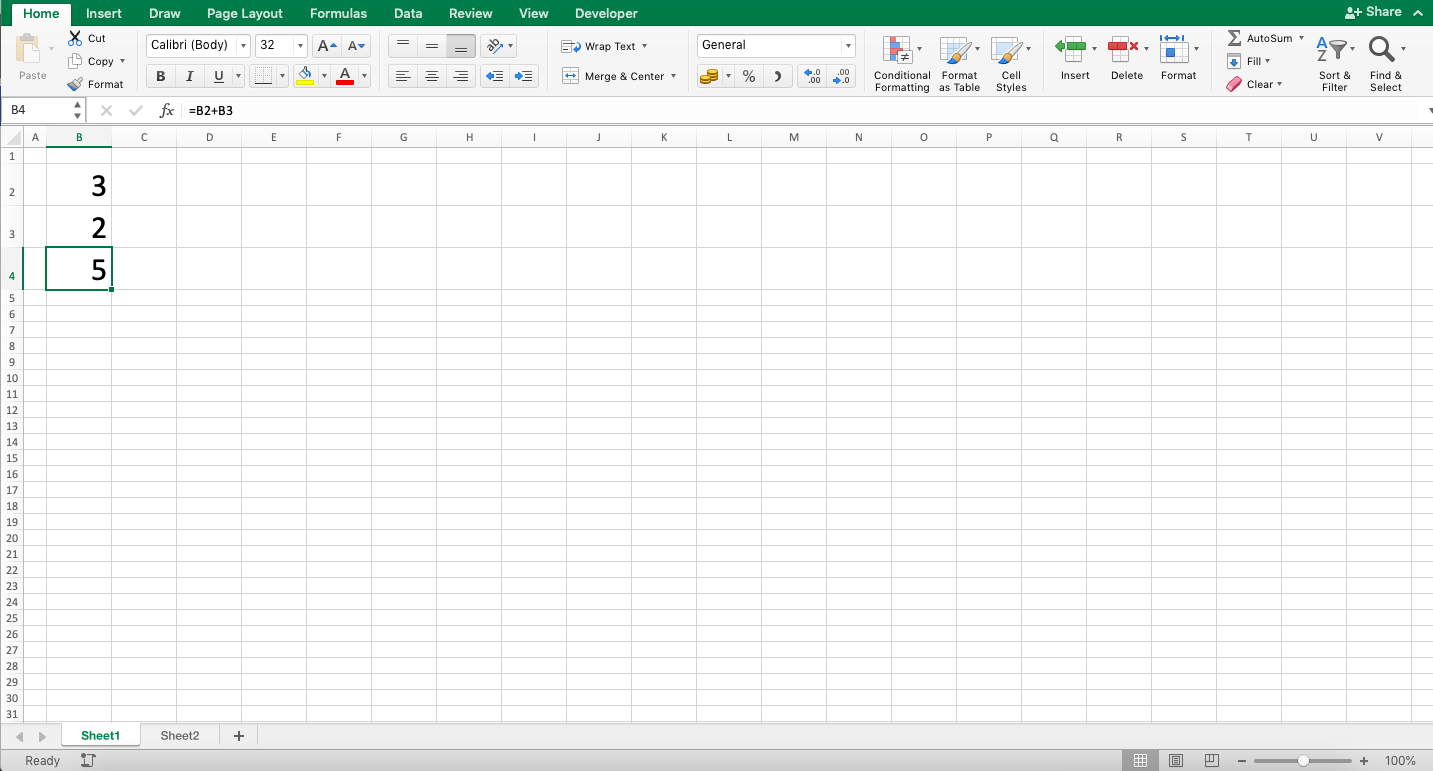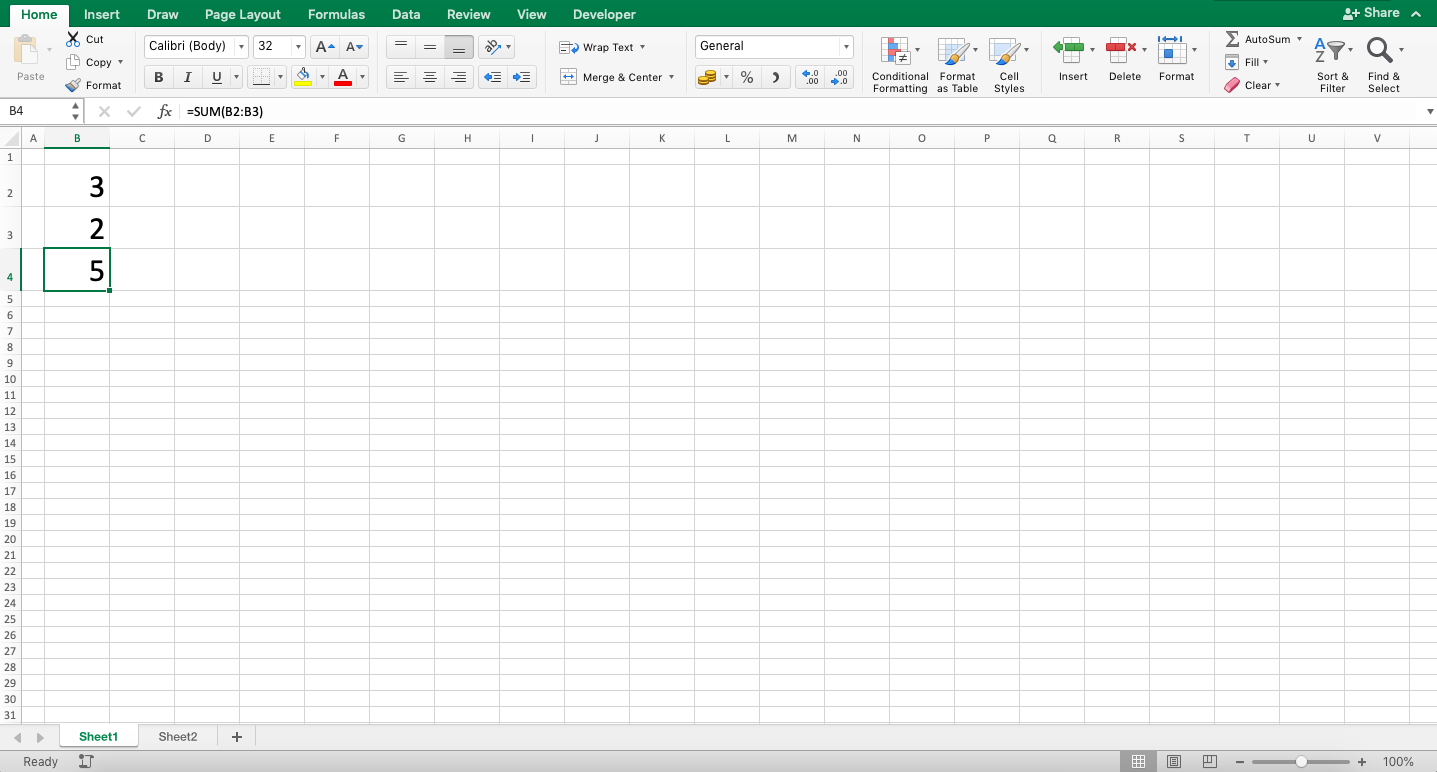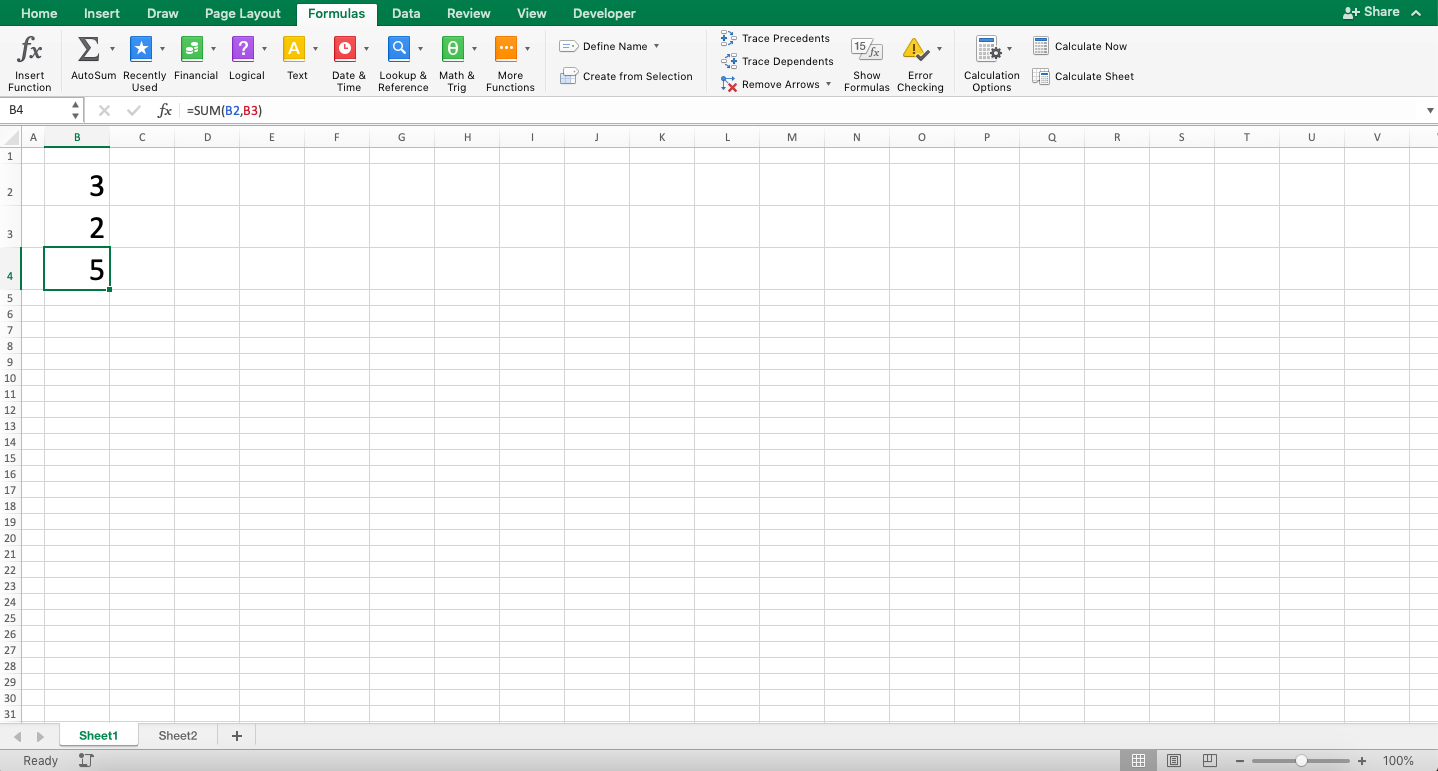How to Write Excel Formula - Compute Expert

# How to Write Excel Formula

Home >> Excel Tutorials from Compute Expert >> Excel Tips and Trick >> How to Write Excel Formula

In this tutorial, we will discuss how to write a formula in excel. Formulas are also often called functions in excel. The understanding of its writing is important so you can utilize this feature to process your data faster and more accurately.

The way to write an excel formula itself is actually pretty simple to understand. This might be what you think also when you have seen its writing steps in the next part. As long as it is often practiced, the writing should become an automatic thing for you when you need it.

## The Ways to Write a Formula in Excel

The following will explain the ways you can use to write an excel formula. Generally, this can be done in three ways:
• Manually (Usually used for mathematics calculation of number data)
• Using formulas provided by excel (Self-writing)
• Using formulas provided by excel (Using the Formula Builder menu)

What are the steps to implement each of these writing methods? Here are the details.

### Method 1: Manually (Usually Used for Numeric Data Calculation)

1. Type an equal sign (=) in the cell where you want to do the writing2. Input the numbers and calculation symbols according to your data processing needs. Here are the calculation symbols usually used in this manual writing method:
• Plus sign (+) is used for addition calculations
• Dash sign (-) is used for subtraction calculations
• Star sign (*) is used for multiplication calculations
• Slash sign (/) is used for division calculations
• Caret sign (^) is used for power and root calculations3. Press Enter
4. Done!### Method 2: Formulas Provided by Excel (Self-Writing)

1. Type an equal sign (=) in the cell where you want to do the writing2. Type the formula name you want to use (can be with large or small letters). Then, type an open bracket sign3. Input the inputs needed by the formula4. Type a close bracket sign5. Press Enter
6. Done!### Method 3: Formulas Provided by Excel (Using the Formula Builder Menu)

1. Highlight the cell where you want to do the writing2. Go to the Formula tab3. Click the Insert Function menu4. Choose by double-clicking the formula you want to use in the dialog box shown5. Input the inputs asked by the dialog box6. Click Done and close the dialog box by clicking the X mark on the top right side of the dialog box7. Done!What do you think? Pretty easy, isn’t it? Use whichever method you prefer and need for your data processing process!

## The Example of the Final Result of a Formula Writing in Excel

After understanding the writing steps above, probably you also want to see the examples of the writing when it is done. The following will give and explain those examples for you.In the example above, we can see how the excel formula writing is done with its result. In using this feature, one thing for sure is you must begin the writing using the equal sign (=).

The manual writing method of a formula can be done by inputting all numbers to calculate and the appropriate calculation symbols. As you can see, the addition process is done by inputting the numbers and plus signs like usually done for summing.In the second example, we can see the writing using the formula provided by excel. There are hundreds of formulas to choose from. You just need to pick the one most appropriate for your needs.

In the formula bar on the screenshot, we can see one of those provided formulas which is SUM. SUM can be used to total the numeric data in excel. The inputs of SUM itself are the numbers you want to total.

The characteristic of writing this method is inputting the equal sign (=) followed by the formula name. Then, input an open bracket sign, the inputs, and a close bracket sign after the inputs are finished being inputted.

Want to input and process data in excel faster? Use the Add-Ins from XLTools! Use our discount coupon code: “7C2L8B31AZ” so you get a 10% discount on us when you purchase its lifetime license!

## The Benefits of Using Formulas

Formulas are very useful to help you in various data processing that you want to do in this software. The use of this essential feature can make your data processing process done faster and more accurately.

## The Writings of Specific Formulas for Your Data Processing Needs

As inferred before, the right formula writing form for your data processing should be specific. The formula to lookup for data and to multiply numbers in excel are obviously different in their writing details.

The writing ways given in here is a general way to write your formula. This is given so you know the big picture of how to write a formula in excel (after all, they have, more or less, a similar pattern of writing).

If you need to learn specific formula writings, visit our tutorial choices page for excel calculations and formulas. There, you just have to choose which tutorial you need to learn the specific writing of!

## Exercise

After understanding the ways to write an excel formula, now is the time to do an exercise. This is so you can deepen your knowledge of how to do the writing!

### Question

Multiply the two numbers in the exercise file using:
2. The formula provided by excel (self-writing). Use PRODUCT to answer this (Hint: the way to use PRODUCT is similar to SUM, which has been given the usage example before!)
3. The formula provided by excel (using the Formula Builder menu). Use the PRODUCT formula too to answer it

The formulas in excel can also be combined in one writing to get the data processing result you need. One of the most popular formula combinations is probably the INDEX MATCH. The combination of INDEX and MATCH can be used to lookup for data much easier and faster.

Want to learn how to use excel faster? Join this popular Udemy course!

Related tutorials you should learn too:

Get updated excel info from Compute Expert by registering your email. It's free!